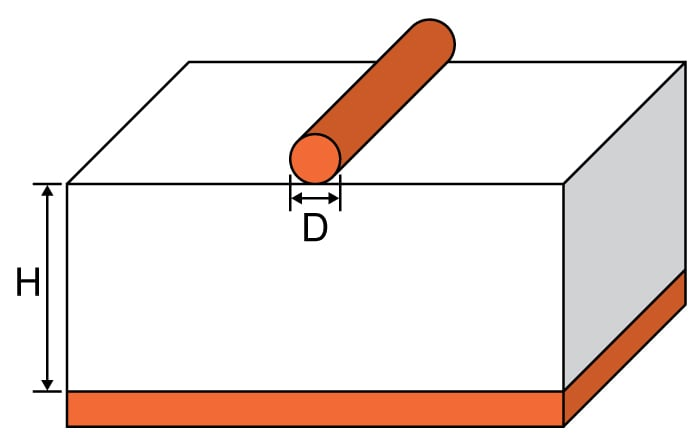# Wire Microstrip Impedance Calculator

## A helpful tool for calculating the impedance of a wire microstrip.

Ohms

### Overview

This tool is designed to compute for the characteristic impedance of a wire microstrip. Such a microstrip is constructed similarly to the standard microstrip but uses a round conductor instead of a flat trace. As with other microstrip devices, the conductor and the ground plane are separated by a dielectric.

To use this calculator, enter the values for the wire diameter and substrate dielectric and press the "calculate" button. The resulting impedance (in ohms) will then be displayed. Note that if the wire is insulated, then the results of this calculator will just be an estimate. Also, take into consideration the board's dielectric thickness and the wire insulation in the height (H).### Equation

$$Z_{0_{wm}}=\frac{\eta_{o}}{2\pi \sqrt{er_{eff}}}\cdot \ln \left ( \frac{2H+D}{D}+\sqrt{\frac{2H+D}{D}\cdot \frac{2H+D}{D}-1} \right )=\frac{\eta_{o}}{2\pi \sqrt{er_{eff}}}\cosh^{-1}\left ( \frac{2H+D}{D} \right )$$

Where:

$$er_{eff1}=\frac{er+1}{2}+\frac{er-1}{2}\cdot \left [ \sqrt{\frac{d}{d+12h}}+.04\cdot \left ( 1-\frac{d}{h} \right )^{2} \right ]$$
$$er_{eff2}=\frac{er+1}{2}+\frac{er-1}{2}\cdot \left[ \sqrt{\frac{d}{d+12h}} \right ]$$

if $$(\frac{D}{H}<1)$$:

$$er_{eff}=er_{eff1}$$
else:

$$er_{eff}=er_{eff2}$$

$$\eta_{o}$$ = wave free space impedance ≈  377 Ω

Note: The above equations are from IPC-2141A "Design Guide for High-Speed Controlled Impedance Circuit Boards"

### Applications

A wire microstrip is used mainly as a transmission line for microwave applications. It is often considered when a standard microstrip is not available. It is also favored over the stripline transmission line, which is constructed with a more expensive printed circuit board style. In fact, a wire over the top of a printed circuit board or copper clad material can be considered a simple wire microstrip.